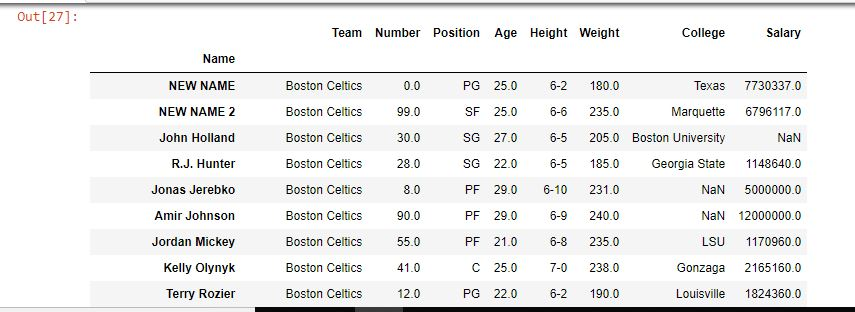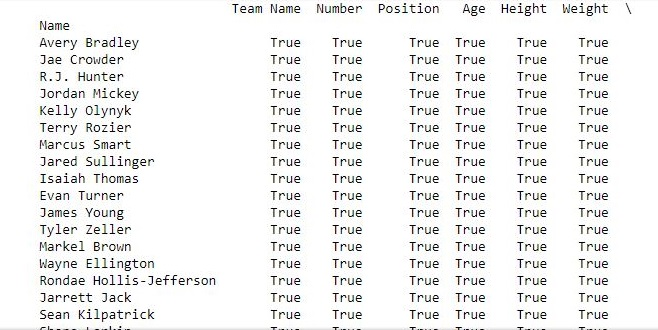Open In App

# Python | Pandas Dataframe.rename()

Python is a great language for doing data analysis, primarily because of the fantastic ecosystem of data-centric python packages. Pandas is one of those packages and makes importing and analyzing data much easier.

Pandas `rename()` method is used to rename any index, column or row. Renaming of column can also be done by `dataframe.columns = [#list]`. But in the above case, there isn’t much freedom. Even if one column has to be changed, full column list has to be passed. Also, the above method is not applicable on index labels.

Syntax: DataFrame.rename(mapper=None, index=None, columns=None, axis=None, copy=True, inplace=False, level=None)

Parameters:
mapper, index and columns: Dictionary value, key refers to the old name and value refers to new name. Only one of these parameters can be used at once.
axis: int or string value, 0/’row’ for Rows and 1/’columns’ for Columns.
copy: Copies underlying data if True.
inplace: Makes changes in original Data Frame if True.
level: Used to specify level in case data frame is having multiple level index.

Return Type: Data frame with new names

Example #1: Changing Index label

In this example, the name column is set as index column and it’s name is changed later using the rename() method.

 `# importing pandas module``import` `pandas as pd`` ` `# making data frame from csv file``data ``=` `pd.read_csv(``"nba.csv"``, index_col ``=``"Name"` `)`` ` `# changing index cols with rename()``data.rename(index ``=` `{``"Avery Bradley"``: ``"NEW NAME"``,``                     ``"Jae Crowder"``:``"NEW NAME 2"``},``                                 ``inplace ``=` `True``)``# display``data`

Output:
As shown in the output image, the name of index labels at first and second positions were changed to NEW NAME & NEW NAME 2.Example #2: Changing multiple column names

In this example, multiple column names are changed by passing a dictionary. Later the result is compared to the data frame returned by using .columns method. Null values are dropped before comparing since NaN==NaN will return false.

 `# importing pandas module``import` `pandas as pd`` ` `# making data frame from csv file``data ``=` `pd.read_csv(``"nba.csv"``, index_col ``=``"Name"` `)`` ` ` ` `# changing cols with rename()``new_data ``=` `data.rename(columns ``=` `{``"Team"``: ``"Team Name"``,``                                  ``"College"``:``"Education"``,``                                  ``"Salary"``: ``"Income"``})`` ` `# changing columns using .columns()``data.columns ``=` `[``'Team Name'``, ``'Number'``, ``'Position'``, ``'Age'``,``                ``'Height'``, ``'Weight'``, ``'Education'``, ``'Income'``]`` ` `# dropna used to ignore na values``print``(new_data.dropna()``=``=` `data.dropna())`

Output:
As shown in the output image, the results using both ways were same since all values are True.